#### Chapter 7 Introduction to Euclid's Geometry R.D. Sharma Solutions for Class 9th Math Exercise 7.1

Exercise 7.1

1. Define the following terms:
(i) Line segment
(ii) Collinear points
(iii) Parallel lines
(iv) Intersecting lines
(v) Concurrent lines
(vi) Ray
(vii) Half-line.

Solution

The given problem requires definitions of various terms.
(i) Line segment: A line segment AB can be defined as the part of the line with end points A and B, where A and B are the two points of the line.
It is denoted by ABor BA
Let us take a line with two points A and B(ii) Collinear points: When three or more points lie on the same line; they are said to be collinear.
For Example: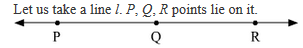So, Here, P, Q and R are collinear points.

(iii) Parallel lines: Two or more lines are said to be parallel to each other if there is no point of intersection between them.
For Example: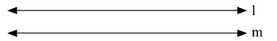Since, there is no point of intersection between l and m, they are parallel.
(iv) Intersecting lines: Two or more lines are said to be intersecting lines if they meet each other at a point or they have a common point.
For Example:l and m are the two lines both passing through point O. Hence, they are intersecting lines.
(v) Concurrent lines: Two or more lines are said to be concurrent if they all pass through a common point or there exist a point common to all of them.
For Example:m, n, o and p are concurrent as they all have a common point O.

(vi) Ray: A ray is defined as the part of the line with one end point such that it can be extended infinitely in the other direction. It is represented by AB
For Example:Here, AB is a ray as it has one end point A and it can be extended indefinitely in other direction.

(vii) Half-line: A half-line can be defined as a part of the line which has one end point and extends indefinitely in the other direction. It is different from ray as the end point is not included in the half-line.
For example:When A is included in the part, then it is called a ray AB, but when A is not included then is called a half-line AB.

2. (i) How many lines can pass through a given point?
(ii) In how many points can two distinct lines at the most intersect?

Solution

(i) Let us take a point A.
If we try to draw lines passing through this point A, we can see that we can draw many lines.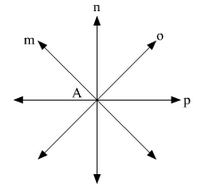Therefore, infinite number of lines can pass through a given point.

(ii) Let us take two lines l and m, and intersect them.As, we can see here the two lines have only one point in common that is O.
Therefore, there is only one point where two distinct lines can intersect.

3. (i) Given two points P and Q, find how many line segments do they deter-mine.
(ii) Name the line segments determined by the three collinear points P, Q and R.

Solution

(i) In this problem we are given two points P and Q.If we try to join these two points through a line segment, we can see that there can be only one such line segment PQ.Therefore, given two points, only one line segment is determined by them.

(ii) In the given problem, we are given three collinear points P, Q and R. Collinear points lie on the same line, so they can be represented as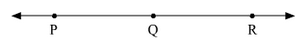So, the various line segments determined here are PQ, QR and PR.

4. Write the truth value (T/F) of each of the following statements:
(i) Two lines intersect in a point.
(ii) Two lines may intersect in two points.
(iii) A segment has no length.
(iv) Two distinct points always determine a line.
(v) Every ray has a finite length.
(vi) A ray has one end-point only.
(vii) A segment has one end-point only.
(viii) The ray AB is same as ray BA.
(ix) Only a single line may pass through a given point.
(x) Two lines are coincident if they have only one point in common.

Solution

(i) False
The given statement is false, as it is not necessary that the two lines always intersect. In the case of parallel lines, the two lines never intersect or there is no common point between them.

(ii) False
The given statement is false, as there is only one point common between two intersecting lines. So, two lines cannot intersect at two points.

(iii) False
A line segment is a part of line defined by two end points, so it is always of a definite length. Hence, the given statement is false.

(iv) True
The given statement is true as a unique line can be determined by minimum of two distinct points.

(v) False
A ray is defined as a part of the line with one end point, where the other end can be stretched indefinitely. Therefore, a ray cannot have a finite length.

(vi) True
The given statement is true as a ray is defined as a part of the line with one end point, where the other end can be stretched indefinitely.

(vii) False
A line segment is a part of line defined by two end points. So the given statement is false as a line segment has two end points.

(viii) False
While denoting a ray, the first letter denotes the end point and the second one denotes the end which can be extended. So, for ray AB, A is the end point and B is the end which can be stretched while for ray BA, B is the end point and A the end which can be stretched. Therefore, ray AB is not the same as ray BA.
(ix) False
The given statement is false as infinite number of lines can pass through a given point.

(x) False
Two lines are said to be coincident if they lie exactly on top of each other, which means that they have all the points in common. So the given statement is false.

5. In Fig. 7.17, name the following:(i) five line segments.
(ii) Five rays.
(iii) Four collinear points.
(iv) Two pairs of non-intersecting line segments.

Solution

(i) A line segment is a part of line defined by two end points. So in the given figure 7.17, five line segments are: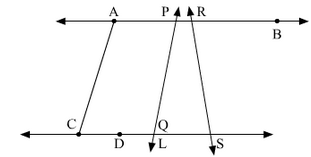(1) AC
(2) CD
(3) AP
(4) PQ
(5) RS

(ii) A ray is the part of line with one end point and one end which can be extended. So in the given figure 7.17, five rays are:
(1) Ray RB
(2) Ray RS
(3) Ray PQ
(4) Ray DS(5) Ray AB

(iii) Collinear points are the points which are present on the same line. In the present figure 7.17, there are two sets of four collinear points.
(1) A, P, R, B
(2) C, D, Q, S

(iv) In the given figure 7.17, two pairs of non intersecting line segments are:
(1) AB and CS
(2) AC and PQ

6. Fill in the blanks so as to make the following statements true:
(i) Two distinct points in a plane determine a ........line.
(ii) Two distinct ........... in a plane cannot have more than one point in common.
(iii) Given a line and a point, not on the line, there is one and only ......... line which passes through the given point and is ......... to the given line.
(iv) A line separates a plane into ......... parts namely the ...........and the ........... itself.

Solution

(i) Two distinct points in a plane determine a unique line.

(ii) Two distinct lines in a plane cannot have more than one point in common.

(iii) Given a line and a point, not on the line, there is one and only perpendicular line which passes through the given point and is perpendicular to the given line.

(iv) A line separates a plain into three parts namely the two half planes and the line itself.